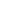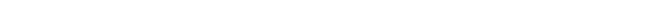The Deviation Between the Theoretical and Empirical Value of Planck’s constant (h) - “From Paradox to Paradigm”## The Deviation Between the Theoretical and Empirical Value of Planck’s constant (h)

When you are interested in physics you must read “Unbelievable“!

Theoretical Physics endorsed the drag coefficient of Fresnel when the empirical “confirmation” Fizeau showed a deviation of 10%!

Is the derived formula for Planck’s constant false when the deviation is just 0.35%?

Statistically it is impossible to obtain coincidence a formula for Planck’s constant with just a deviation of 0.35%. Scientists should acknowledge that and let the scientific debate determine whether the theory behind the derivation is acceptable or not. Rejection of manuscripts science and physic journals, that reveal serious omissions in the past, only on the argument that the article is not relevant or not actual reveals incompetence.

When editors and referees are convinced the discovered omissions are of no consequence for the present perception QM of physic processes they still should not reject papers on bogus arguments. It is the task of the scientific community to determine that. An editor and/or referee do not represent this community. A debate, whether the new perspectives should be rejected or not, is the proper scientific process. Rejection of articles editors or referees based on invalid arguments degenerates science and scientists.

Although this paper reveals more than enough arguments and evidence to justify publication without explaining the deviation of 0.35% I will indicate how the deviation can be explained. In the article *** marks the formulas where the theoretical and experimental values deviate factor 1.003458. It appears that the deviation factor of 1.003458 is systematic. The apparent cause for the deviation implies a difficult mathematical problem that far exceeds my capabilities.

The empirical value of h is obtained with the formula that describes the relation between the energy and the frequency of the photon; E=hv. The derivation of theoretical formula for the Planck-distance (12) is based on this equation. Other formulas refer to particles with mass.

The photon propagates through space and does not distort tense free ether. Masses however distort the surrounding ether. A nucleus, a charged mass, affects the stress free cubical orientated ether (fig. 2) and shapes the surrounding space into a tensed spherical orientation. The stress free cubical orientated space contains more point-volumes or ether per volume than spherical orientated ether surrounding the nucleus of an atom. The packing density of point-volumes in the spherical orientated space/ether around a nucleus therefore differs from the cubical tense free ether packing density. (figures 2,3 and 5).

When a nucleus polarizes the surrounding ether/space (electric field) the point-volumes are forced to orientate into a spherical shape. The same number of point-volumes around a charged nucleus occupy more space. The experimental determined constant of Planck and the formula for the Planck-distance refer to physics of a tense free cubical orientated ether. While deriving Planck’s constant the difference between stress and stress-free ether is not mathematically addressed. The packing difference possibly explains the systematic deviation factor of 1.003458.

Editors, referees and other scientists should address this possibility first before disqualifying the ether theory only based on the argument that the theoretical value of Planck’s constant differs slightly from the experimental value and is therefore false.

Next chapter: The Quantisation of Physics Means of the Quantum DistanceAutomated page speed optimizations for fast site performance